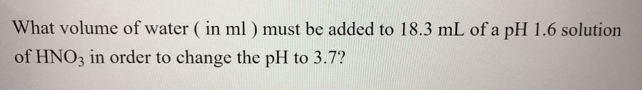Chemistry Practice Problems Strong Acid-Base Calculations Practice Problems Solution: What volume of water (in ml) must be added to 18.3...

# Solution: What volume of water (in ml) must be added to 18.3 mL of a pH 1.6 solution of HNO3 in order to change the pH to 3.7?

###### Problem

What volume of water (in ml) must be added to 18.3 mL of a pH 1.6 solution of HNO3 in order to change the pH to 3.7?View Complete Written Solution

Strong Acid-Base Calculations

Strong Acid-Base Calculations

#### Q. Calculate the pH and the pOH of an aqueous solution that is 0.035 M in HCl (aq) and 0.060 M in HBr(aq) at 25 C.

Solved • Thu Apr 19 2018 16:54:59 GMT-0400 (EDT)

Strong Acid-Base Calculations

#### Q. What is the pH of 8 x 10 -8 M HCl?

Solved • Mon Mar 26 2018 11:20:14 GMT-0400 (EDT)

Strong Acid-Base Calculations

#### Q. For each of the following strong base solutions, determine [OH-], [H3O+], pH, and pOHa. 8.77 x 10-3 M LiOH  b. 1.15 x 10-2 M Ba(OH)2  c. 5.3 x 10-4 M ...

Solved • Tue Mar 13 2018 11:59:59 GMT-0400 (EDT)

Strong Acid-Base Calculations

#### Q. For the following strong base solution, determine [OH-], [H3O+], pH, and pOH. 0.20M NaOH

Solved • Mon Mar 12 2018 14:52:07 GMT-0400 (EDT)